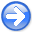PreconditioningNext: EMPTY BINS AND PRECONDITIONING Up: Preconditioning Previous: Blocky models

# INVERSE LINEAR INTERPOLATION

data
Figure 7.
The input data are irregularly sampled.The first example is a simple synthetic test for 1-D inverse interpolation. The input data were randomly subsampled (with decreasing density) from a sinusoid (Figure 7). The forward operatorin this case is linear interpolation. We seek a regularly sampled model that could predict the data with a forward linear interpolation. Sparse irregular distribution of the input data makes the regularization enforcement a necessity. I applied convolution with the simpledifference filter as the operatorthat forces model continuity (the first-order spline). An appropriate preconditionerin this case is recursive causal integration.conv
Figure 8.
Convergence history of inverse linear interpolation. Left: regularization, right: preconditioning. The regularization operatoris the derivative operator (convolution with. The preconditioning operatoris causal integration.As expected, preconditioning provides a much faster rate of convergence. Because iteration to the exact solution is never achieved in large-scale problems, the results of iterative optimization may turn out quite differently. Bill Harlan points out that the two goals in (15) conflict with each other: the first one enforces ``details'' in the model, while the second one tries to smooth away the details. Typically, regularized optimization creates a complicated model at early iterations. At first, the data-fitting goal (15) plays a more important role. Later, the styling goal (15) comes into play and simplifies (smooths) the model as much as needed. Preconditioning acts differently. The very first iterations create a simplified (smooth) model. Later, the data-fitting goal adds more details into the model. If we stop the iterative process early, we end up with an insufficiently complex model, not an insufficiently simplified one. Figure 8 provides a clear illustration of Harlan's observation.

Figure 9 measures the rate of convergence by the model residual, which is a distance from the current model to the final solution. It shows that preconditioning saves many iterations. Because the cost of each iteration for each method is roughly equal, the efficiency of preconditioning is evident.

schwab1
Figure 9.
Convergence of the iterative optimization, measured in terms of the model residual. The ``p'' points stand for preconditioning; the ``r'' points, regularization.PreconditioningNext: EMPTY BINS AND PRECONDITIONING Up: Preconditioning Previous: Blocky models

2015-05-07